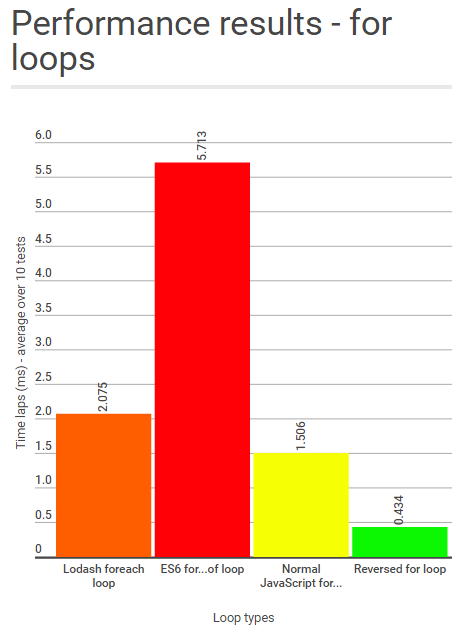Modified on 8th July 2016 following Kevin Farrugia - @imkevdev - and John-David Dalton's review - @jdalton

With Javascript, we are often offered multiple possibilities when it comes to looping if we decide to use libraries. But what are the impacts of each of them on the performance of our script? Let's find it out!

Goal

The objective of this experiment is to test the different performances of the Lodash’s foreach loop, the ES6 for...of loop, the traditional for loop and the improved one, that is to say a reversed loop.

We are going to test these loops on Chrome with the following JavaScript code, that we'll complete depending on the test:

var t0 = performance.now();
for (var p = 0; p < 1000; p++){
// insert the code to test below
// test code finished here
}
var t1=performance.now();
console.log("Took: "+(t1-t0)+"msecs");

It's very simple, but the aim here is to test the performance on a large amount of loops to get reasonable test data. All of the tests we're doing are based on an array of numbers going from 1 to 10. On each recursion, a simple value assignment is done. The array has a length of 10, and the loop iterates for 1,000 times. So each execution of this code represents 10,000 operations.

In the following sections, you'll find the different library imports and JavaScript for each method, the results of the tests appear at the end of this blog article.

Lodash’s foreach loop

Lodash is a JavaScript library that comes from Underscore, the "JavaScript library that provides a whole mess of useful functional programming helpers". First, let's import the lodash library:

<script src="https://cdn.jsdelivr.net/lodash/4.13.1/lodash.min.js"></script>

Our tests will be based on an array of numbers from 1 to 10 as mentioned before. To go over this array, we use the each method that is included in the lodash library.

script.js

var t0 = performance.now();
var tmp;
for (var p = 0; p < 1000; p++){
tmp = value;
});
}
var t1=performance.now();
console.log("Took: "+(t1-t0)+"msecs");

ES6 fOR...OF loop

On the very same basis, we use the for...of loop (known as a foreach loop in most other languages) that is provided by ES6.

script.js

var t0 = performance.now();
var tmp;
for (var p = 0; p < 1000; p++){
tmp = value;
}
}
var t1=performance.now();
console.log("Took: "+(t1-t0)+"msecs");

For loop - Vanilla JavaScript

This time, we are not implementing a for-each loop, but a normal for-loop. That is to say we assign a variable going over the indices of the array.

var t0 = performance.now();
var tmp;
for (var p = 0; p < 1000; p++){
for(var i = 0; i<someNumbers.length; i++){
}
}
var t1=performance.now();
console.log("Took: "+(t1-t0)+"msecs");

Reversed loop - Vanilla JavaScript

The reversed loop is an improved variation of the for-loop, as the length of the array is calculated only one time.

var t0 = performance.now();
var tmp;
for (var p = 0; p < 1000; p++){
for(var i = someNumbers.length-1; i>=0; i--){
}
}
var t1=performance.now();
console.log("Took: "+(t1-t0)+"msecs");

Conclusion

After having performed these tests 10 times each, we get 4 time lap averages, one per technology, that can be summed up in this chart:We can clearly see on this graph that "foreach" loops are slower than normal loops and that Lodash is more efficient than ES6 when it comes to looping. Vanilla JavaScript reversed for-loops are still the most efficient though.

So it turns out when there is a lot of data to treat, the best thing to do would be to go for a simple reversed loop. You may think that the difference is really slight, but the reversed for-loop is still 13 times faster than the ES6's for-of loop. When there is a lot of data to treat, these minor improvements could save your users a considerable amount of time.